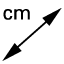# Is Bermuda One?

## Definition 20-21

Of trilateral figures, an equilateral triangle is that which has its three sides equal, an isosceles triangle that which has two of its sides alone equal, and a scalene triangle that which has its three sides unequal. We define triangles in two ways, by their sides and by their angles. When we define triangles by their sides (or legs) we are comparing how many sides are equal: equilateral (equal lines) triangle: three equal sides isosceles (balanced legs) triangles: two equal sides scalene (unequal) triangle: no equal sides Further, of trilateral figures, a right-angled triangle is that which has a right angle, an obtuse-angled triangle that which has an obtuse angle, and an acute-angled triangle that which has its three angles acutes. The second way we define triangles is by their angles and what the defining angle is in the triangle: right triangle: contains one right angle (and two acute angles) obtuse triangle: contains one obtuse angle (and two acute angles) acute triangle: contains three acute angles

## Tool Practice

I have given you (temporarily) a new tool, which you can use to measure side lengths of shapes by clicking on them. Using the figures you have been provided below, see if you can identify each triangle type. HINT: Triangles can be labeled by their sides or by their angles, so a right triangle must also be either scalene or isosceles (why can't a right triangle be equilateral?) so each triangle will have two names. Move the points around and see if it changes your labels.